Thank you for visiting nature.com. You are using a browser version with limited support for CSS. To obtain the best experience, we recommend you use a more up to date browser (or turn off compatibility mode in Internet Explorer). In the meantime, to ensure continued support, we are displaying the site without styles and JavaScript.

# On the thermodynamically stable amorphous phase of polymer-derived silicon oxycarbide

## Abstract

A model for the thermodynamic stability of amorphous silicon oxycarbide (SiCO) is presented. It builds upon the reasonably accepted model of SiCO which is conceived as a nanodomain network of graphene. The domains are expected to be filled with SiO2 molecules, while the interface with graphene is visualized to contain mixed bonds described as Si bonded to C as well as to O atoms. Normally these SiCO compositions would be expected to crystallize. Instead, calorimetric measurements have shown that the amorphous phase is thermodynamically stable. In this article we employ first-principles calculations to estimate how the interfacial energy of the graphene networks is favorably influenced by having mixed bonds attached to them. We analyze the ways in which this reduction in interfacial energy can stabilize the amorphous phase. The approach highlights how density functional theory computations can be combined with the classical analysis of phase transformations to explain the behavior of a complex material. In addition we discover a two-dimensional lattice structure, with the composition Si2C4O3 that is constructed from a single layer of graphene congruent with silicon and oxygen bonds on either side.

## Introduction

The interaction between carbon and amorphous silica has been of long-standing interest to scientists from different communities. In the early 1950s attempts were made to introduce carbon into SiO2 for the purpose of enhancing the thermal and electrical conductivity of the silica glass1. These efforts, which entailed stirring graphite into molten silica, did not succeed because carbon is essentially insoluble in silica.

However, more recently, it has been shown that large mole fractions of carbon can be incorporated into compounds of silicon, carbon and oxygen if they are synthesized via the chemical route2. For example when methyltriethylsiloxane, where silicon is coordinated with three oxygen atoms and one methyl (-CH3) group3, is heated to 800–1000 °C in an inert atmosphere, then the methyl group dissociates into hydrogen, which is released to atmosphere, while carbon, bonded to silicon, along with the three O-Si bonds, are retained in the solid state, yielding a compound with an overall Si:C:O composition of approximately 1:1:3. These compounds are amorphous when examined by Bragg diffraction.

The carbon content in these materials, processed from the polymer route, can be varied over a wide range by changing the composition of the organic precursors4. They have come to be known, in general, as polymer-derived ceramics or PDCs. Until recently SiCO materials prepared in this way were thought to be random networks of Si, C and O, which contradicted the fact that silica and carbon are practically insoluble in one another.

Insights into the molecular structure of PDCs began to emerge from the following observations: (i) Although devoid of wide-angle X-ray diffraction peaks, the SiCO materials exhibit strong signals in Small Angle X-ray Scattering (SAXS) suggesting the presence of short range order5, (ii) Strong D and G peaks in Raman spectroscopy suggested the presence of extended states of sp2 carbon within the structure6, (iii) NMR measurements of 13C confirmed the presence of sp2 carbon, but, in addition, the 29Si spectra showed the presence of mixed bonds of SiC4-nOn for n = 1, 2 and 37 and (iv) Measurements of creep at temperatures above 1300 °C showed the remarkable result that the materials exhibited viscoelastic behavior, but not viscous flow as occurs in silica glass8. These observations, taken together, led to a nanodomain model for the molecular structure which is shown in Fig. 1(a)9. The model shows three-dimensional network of graphene sheets forming a network of nanodomains that contain within them silicon-oxygen molecules. The mixed bonds between Si, C and O lie at the interface with the graphene walls. In the model, the size of the nanodomains is calculated to be 1–5 nm, which agrees with the SAXS measurements9. The model not only explains the SAXS, Raman and NMR data but is also consistent with the creep resistance at high temperatures. Viscous flow is prevented by the graphene network bearing the applied load as the silica molecules within the domains relax; as such the material shows viscoelastic behavior but not steady state flow.

The validity of the graphene network model has been confirmed by molecular dynamics calculations10, as well as by first principles DFT calculations11. One such result is given in Fig. 1(b). It shows the segregation of carbon into graphene and the formation of mixed bonds between it and silicon-based molecules. Other descriptions12,13 of the segregation of carbon in SiCO are essentially consistent with9; however only the latter work presents quantitative predictions of the domain size (as a function of the carbon content) which compare favorably with the measurements by SAXS.

The most remarkable recent development has been measurements of the enthalpy of formation of SiCO compounds by high temperature calorimetry which asserts that the amorphous state of these materials has a lower enthalpy than the crystalline state14. We seek to explain the stability of the amorphous phase of silica in the nanodomain model of Fig. 1(a), in terms of the energy of the graphene-SiO2 interface, which is calculated from first principles.

## Framework for analyzing the thermodynamic stability of the amorphous phase

We seek to determine whether or not the amorphous phase of silica in the nanodomain model shown in Fig. 1(a) can be stabilized by the interfacial energy that it forms with graphene, via the mixed bonds between carbon, silicon and oxygen. If the amorphous phase were to crystallize then these mixed bonds will not survive, effectively increasing the interfacial energy in the nanodomain structure. If the domain size is very small, with a high interface to volume ratio, then the interfacial penalty would dominate the gain in the volumetric free energy, thereby stabilizing the amorphous network.

We follow the hypothesis that the mixed bonds of Si present at the graphene interface lowers its energy. These mixed bonds are silicon-centered tetrahedra consisting of Si bonded to oxygen on one side and the carbon atoms in graphene on the other. These bonds are the source of interfacial bonding and a lower interfacial energy. We estimate the change in the interfacial energy in terms of the cost of breaking C-Si bond in these mixed bond tetrahedra.

The unusual feature of the C-Si bonds is that they force the carbon atoms in graphene to change their hybridization from sp2 to sp3; thus the C-Si bond induces strain in the adjacent C-C bonds which leads to a minimum in the interfacial energy when calculated as a function of the density of Si bonds on the graphene face.

The graphene segments in the nanodomain structure would contain edges with the spatial frequency of the nanodomain size. The edges are defect sites where the mixed bond interactions will be stronger. We shall consider both the face and the edge coordinations of the C-Si-O mixed bonds at the interface. In this section a framework for this analysis is developed which can lead to a quantitative estimate of the domain size which can be stabilized in this way.

### Free Energy considerations for amorphous to crystal transformation

The argument for the thermodynamic stability of the amorphous nanodomain structure of SixCyOz, as determined from high temperature calorimetric measurements14, centers on the functionalization of graphene surfaces and edges with mixed-bonds. Crystallization would require the mixed-bonds to be broken.

The mixed-bonds are attached to graphene in two ways: on the face of the graphene segments or along its edges as shown schematically in Fig. 2. We write the change in Gibbs free energy between the crystalline state (called State I) and the amorphous state (State II), by the quantity ΔGII-I. We assume that the mixed bonds are present in the amorphous state but broken in the crystalline state, that is, the interfacial energy of graphene, with the mixed bonds attached to it is lower than without the mixed bonds. ΔGII-I is then given by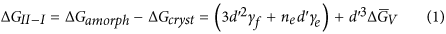where d′ is the size of the nanodomains. Here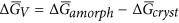is the difference in the free energy of the amorphous and the crystalline state; it is a positive quantity since the crystalline phase has a lower free energy. The bar placed above these free energy quantities implies that they have units of energy per unit volume.

The differences in the interfacial energy of the amorphous state and the crystalline state are written as γf and γe, where the subscript f refers to the graphene face and e to the graphene edges. They are equal to the energy of the bonded state where silicon forms mixed bonds with the carbon atoms in graphene, minus the energy of the separated state where the silicon-carbon bonds have been broken. Defined in this way, both are negative quantities.

The pre-factors in the interfacial energy terms, in Eq. (1), reflect the sharing of interfaces and the edge atoms between adjacent domains, which are, for simplicity, assumed to be in the shape of cubes with an edge length of d′. Each face of the cube is shared by two adjacent domains, thus overall three of such faces belong to one cube, hence the pre-factor of three in the d2γf term. The other interface term refers to the edges of the graphene segments that form the cube. Here there are different possible values for the pre-factor. At one extreme it can be assumed that there is only one edge that is shared by the four cubes that meet there, in which case each edge would be shared between four cubes; since there are twelve edges, three of them would belong to a cube. For this case the pre-factor ne = 3.

It is however, difficult to imagine the edges of the graphene from four adjacent cubes meeting to form a one line of a coherent carbon structure. It is more likely that segments of graphene form the faces of the cubes, each with their own edges that do not dovetail with the edges from the orthogonally aligned adjacent faces. In this instance four edges are shared by the four cubes that meet there and the total number of edges per cube becomes ne = 12. Thus a pre-factor three would be the minimum value for neand twelve its highest possible value. In the following analysis we compute the predictions for the model for ne = 3 → 12.

The form of Eq. (1) when plotted against d′, exhibits a minimum. Its position is analyzed by differentiating the right hand side with respect to d′ and setting the result equal to zero. The solution to this equation gives the value for d′ where the total free energy reaches its minimum value. We call it d*.

Differentiating the right hand side of Eq. (1) gives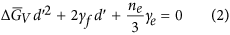This quadratic equation has two solutions. One of them, which is necessarily negative, is not admissible. The viable solution for Eq. (2) gives the following value for the position of the minimum in the free energy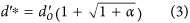where,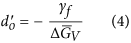and,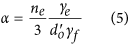Note that in Eq. (4)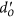is a positive quantity since γf < 0. The quantity in Eq. (5), α, is positive since both γe and γf are negative quantities.

A physical interpretation of the right hand side of Eq. (3) is gained by recognizing that, given by Eq. (4), depends only on the interfacial energy of graphene faces, while α recognizes the influence of edge energy. If the edge energy goes to zero, that is, there are no mixed bonds present at the edges, then α → 0 and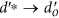. Thus α represents the contribution of the edge mixed bonds to the calculation of the domain size where the free energy. Note that a larger domain size implies that crystallization becomes more difficult, or, in other words, the interfacial energy opposition to crystallization grows stronger.

We now normalize the variables in Eq. (1) into non-dimensional forms. The left hand side of Eq. (1) is the total change in energy. We normalize it with respect to the product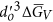, which has units of energy, sincehas units of energy per unit volume. Dividing both sides by this product and substituting forfrom Eq. (4) and for α from Eq. (5) into the terms on the right hand side of Eq. (1) leads to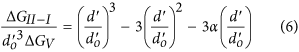Plots of Eq. (6) for different values of α = 0.25 to 2.0 are shown in Fig. 3. The minimums become deeper and move towards a large size of the nanodomains as α increases, reflecting the increasing role of the edge bonds. The values for α are derived from first principles calculations, as described in the next section.

### The significance of mixed interface bonds at graphene edges

In order to determine the relative significance of the mixed bonds formed at the graphene face and the graphene edges, we consider the case where the only contribution to interfacial energy is provided by the mixed bonds present at the edges, without any mixed bonds being present on the face atoms.

For this purpose we omit the face energy term from Eq. (1). Retaining only the edge term gives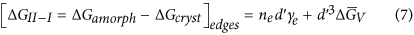Differentiating and equating to zero gives the following result for the domain size when it is stabilized solely the interfacial energy of the graphene edges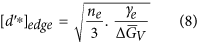### Relating bond-energies to interfacial energies

In the next section we compute the magnitude of the binding energy of the mixed bonds to the face atoms and to the edges of graphene. The interfacial energy depends not only on the energy per bond, but also on the concentration of the Si-C bonds, defined as the number of Si-C bonds as a fraction of the total number of carbon bonds on the surface. For example if one out of ten carbon atoms on the surface contains a Si-bond then the concentration is given by the Si/C ratio is equal to 0.1. Since the binding energy of one mixed-bond is distributed over several carbon atoms, the gain in energy per carbon atom is equal to the product of the binding energy of one bond times the concentration of the bonds. For example if the binding energy of one Si-bond is a quantity X then the binding energy per carbon atom, for a Si/C ratio of 0.1, would be 0.1X. We call this latter quantity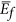for the graphene face andfor the edges.

The interfacial parameters are related toand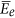in the following way. The average interatomic spacing of carbon atoms in graphene, α, may be set equal to a3 = Ω, where Ω is the effective volume of carbon atom in graphite. Then the following relationships apply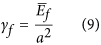and,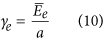The effective volume per carbon atom is Ω = 0.0095 nm3, assuming the density of graphite to be equal to 2.09 g cm–3, gives a = 0.21 nm. Note that γf being face energy has units of energy per unit area while γe has units of energy per unit length.

## First principles computations

### The method

The first-principles calculations were performed using density functional theory (DFT) and plane-wave projector augmented-wave method15 with the exchange-correlation of Perdew-Burke-Ernzerhof16 as implemented in the VASP code17. An energy cutoff of 400 eV was used. The atomic forces were relaxed to be less than 0.03 eV Å−1.

The binding energy of a Si bonded to the graphene surface as a function Si-C bond concentration was calculated by using supercell approximation. The supercell consists of 60-atom graphene with 1–16 SiH3 molecules or 1–8 Si(OH)3 molecules attached to it. The graphene sheets were separated by 20 Å vacuum in the supercell. For the edges both armchair and zigzag configurations were considered.

### A two-dimensional lattice between graphene and silicon dioxide

When exploring the various geometries for attaching Si-O molecules to graphene surfaces, the periodic structure, shown in Fig. 4, was discovered. The average composition of this 2-D structure is Si2C4O3, that is there are two carbon atoms and 1.5 oxygen atoms for every silicon atom. The mixed bond molecules, per silicon atom, have the composition SiC1/4O3/2, with the silicon being coordinated to one carbon and three oxygen atoms. Thus one half of all carbon atoms change their electronic structure from sp2 to sp3 in order to form a bond with silicon. The binding energy per Si bond is calculated to be −1.2 eV. Therefore the quantityfor this structure, according to the definition in the previous section, is equal to −0.6 eV since the concentration of mixed bonds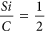. Substituting this value into Eq. (9) gives a value of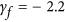J m–2. This would be the work required per unit area to separate the Si-O bonds from the graphene (the separated state is the zero energy state). This magnitude of this interfacial energy is very high (for example the energy of grain boundaries in ceramics, which is also the work of fracture for separating the boundary, are typically about 1 J m–2). The implication is the the 2-D structure shown in Fig. 4 is energetically highly favored.

The question arises whether it is possible to synthesize this 2D lattice. The chemical route to the synthesis of silicon-oxycarbide is likely to yield a distribution of different kinds of interface structures, some of whom may indeed have the arrangement shown in Fig. 4. Calculations for the band gap for this structure gives a value of ~2.5 eV. Optical measurements of the absorption edge18 indeed give similar values for the band gap in silicon-oxycarbide and related materials.

### Binding energy of Si-based molecules to face atoms of graphene

We have conducted approximate calculations for the binding energy of mixed bonds of Si to graphene for the case where Si is bonded to one carbon and three oxygen atoms. The highly covalent nature of the Si-C bond and the singular sp3 nature of the Si orbitals is expected to yield reasonable values for the bonding energy of the Si-C bond when the valency of the remaing three orbitals is satisfied by connecting them to OH or to -H atoms. The energy of the Si-C bond is calculated for both –OH and –H configurations and is found to be essentially similar for both of them, providing evidence for the local nature of the Si-C bond.

The calculated change inwith the concentration of Si bonds,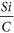, is shown in Fig. 5. Note that at firstdeclines linearly with concentration, but only up to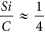. Then it begins to rise, reaching nearly zero when there is one silicon for every two carbon atoms. As shown in the molecular diagram, the sp3 Si-C bond pulls the carbon atoms upwards from the graphene plane, thereby inducing strain in the neighboring carbon atoms. The strain extends to the first nearest carbon. Thus if two silicon bonds were to be brought closer than the spacing between two nearest neighbor carbon atoms then the strain fields will overlap, thereby placing a penalty on the binding energy of the Si molecule to the graphene layer. (Note that the elastic strain energy is proportional to the square of the strain. Therefore the sum of the square of two equal quantities of strain is only one half of the square of twice that quantity of strain.) In summary, the energy does indeed decline linearly up to the point that silicon atoms are spaced two carbon atoms apart, but then rises when the strain fields begin to overlap.

The magnitude ofreaches a minimum value of −0.2 eV at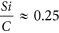. Substituting this value in Eq. (9) and recognizing that a = 0.21 nm, gives that γf = −0.76 J m–2. As a comparison the typical value for the grain boundaries in ceramics is ~1 J m–2. The grain boundary energy is defined as the work that would be required to separate the boundary to free surfaces. A similar definition is used for γf, that is, it is the work needed to break the silicon-carbon bond to create two free surfaces.

In the above discussion we have considered mixed bonds of the SiC1/4O3/2 chemistry, that is, where Si is coordinated to one carbon (in the graphene) and three oxygen atoms. Attempts to model a situation where one silicon is bonded to two carbon atoms on the graphene face were unsuccessful, yielding very weak bonds. However, strong interaction was obtained if a carbon atom in the graphene face was removed creating a carbon-vacancy and the Si was inserted into the vacancy to form three bonds with carbon, with the one Si-O bond lying normal to the graphene plane. In this instance a very high value of the binding energy (−3.14 eV) between the Si and graphene was obtained. If these bonds do indeed form while the polymer is converting into the ceramic then their influence on the interfacial energy and thereby on the stability of the amorphous phase is likely to be profound.

In the following section we analyze the bonding of Si-O molecules to carbon atoms placed at graphene edges. The edge energy, γe is a line energy and would be equal to the work required to separate the interface (please see Fig. 5) along the edge of graphene.

### Binding energy of Si-based molecules to the edge atoms of graphene

The edges of graphene consist of dangling bonds. Therefore the intrinsic energy of the edges is much higher than of graphene surfaces. The Si mixed bonds attached to the carbon atoms at these edges can produce a significant reduction in energy. Results from these simulations where Si atoms bond to one or to two carbon atoms at edges of either zigzag or armchair configurations are shown in Fig. 6. The energy associated with these configurations are summarized in Table. 1.

There is no perceptible strain associated with the Si bonds attached to the edges, which stands in contrast to the Si atoms bonded to the face atoms of graphene. Therefore the (magnitude of the) interfacial energy is likely to rise linearly with the number concentration of Si atoms present at the edges. However, visual inspection of the molecular configurations suggests that steric hindrance will prevent the Si atoms being closer than approximately 4 carbon atom-spacings for the single Si-C bonds and 8 carbon atom-spacings for the double Si-C bonds. Substituting the calculated values for the binding energy of the Si mixed-bonds into the relationship forand γe, from Eq. (10), gives the values for the edge interfacial energy. The values for γe obtained in this way for the four geometries of the graphene edges are listed in Table 1. They range from 0.54 × 10–10 J m–1 to 0.77 × 10–10 J m–1, with the lowest value corresponding to the Si-1C, zig-zag configuration and the highest to the Si-2C, zig-zag configuration, as given in Fig. 6. Here 1C and 2C implies that the Si is bonded to one or two carbons at the graphene edge.

### Comparison with experiments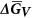It is possible to seek a comparison between the measurements of the average size of the nanodomains in the amorphous structure of SiCO, measured by SAXS, which have been reported in ref. 9 and their value predicted from above analysis. The prediction depends on two quantities, the difference in the Gibbs Free energy between the crystalline and the amorphous states,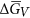and the interfacial energies.

The high temperature calorimetric measurements14 provide the difference between the enthalpy of the amorphous and the crystalline state, that is,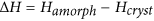. The free energy is given by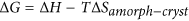. Since the amorphous phase has a higher entropy than the crystalline phase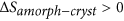and therefore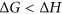. Thus the free energywould be somewhat less than the difference in enthalpy. In the present analysis we are overestimating the free energy by assuming that it is equal to the enthalpy measured in the calorimetry experiments14.

Below we estimate the size of the nanodomains assuming that the free energy is equal to the enthalpy measured from calorimetric experiments. It is immediately evident from Eq. (4) that an overestimate of the free energy would lead to an underestimate of the size of the nanodomains. Thus the actual values of the domain size are likely to be greater than the calculated values, given below, by the same fraction that we are overestimating the free energy.

The procedure and the equations that lead to the comparison between theory and experiment are outlined in Fig. 7. The interfacial energies, γf and γe are obtained from the computed values forandusing Eq. (9) and (10). We use the lowest (the most negative) values of γf and γe for comparison with experimental data. The lowest value for γf, as calculated above is −0.76 J m–2 and the lowest value for γe is −0.77 × 10–10 J m–1.

The enthalpy of the amorphous state relative to the crystalline state was reported in ref. 14 in units of kJ mol–1. It was converted to, which has units of J m–3, by calculating the molar volume of SixCyOz from its composition and assuming a density of 2 g cm–3 (the values for the density of SiCO range from 2.0 to 2.2 g cm–3 19).

The values for γf, γe andmay now be inserted into Eq. (4) to calculate, from Eq. (4) and α from Eq. (5). These values can now be used to obtain the theoretical estimate of the domain size, d*, from Eq. (3). In this case the interfacial energy includes the mixed bonds at the faces and the edges of graphene.

The prediction for the domain size by considering only the bonds at the edges and ignoring the bonds on the faces of the graphene segments, is given by Eq. (8).

As had been discussed earlier the number of graphene edges per cube, ne, can range from 3 to 12. Thus we consider these two limiting values for estimating the domain size. The larger value of ne would be expected to lower the interfacial energy further thereby rendering greater stability to the amorphous phase, which would then translate in to a larger value for the domain size.

The high temperature calorimetry data14 for five compositions of SixCyOz, written as S1-S5, are summarized in Table 2. The values forare given in a column on the right hand side. Note that they range from 0.37 to 0.96 nm. The value 0.05 nm for S5 is an outlier, principally because the free energy measurement for this composition was abnormally high. Recall from Eq. (3) that the domain size is predicted to be equal towhen α = 0, that is in the absence of edge bonds. The experimental values of the domain size that usually lie in the 1–3 nm range, are significantly larger than estimated from, implying that the edge mixed-bonds indeed make a significant contribution to the stability of the amorphous phase.

The results from the calculation that includes both the face and the edge mixed-bonds are given in Table 3. The theoretical predictions for ne = 3 and ne = 12 are given by d* in the second column. The theoretical value for the domain size which considers only the edge bonds, [d*]edge, are given in the third column. The right hand column gives the experimental values of the domain size four the specimens S2–S5, as measured by small angle scattering and reported in ref. 9. Disregarding S5, which appears to be an outlier because of its anomalously large value for, the agreement between theory and experiment is remarkably good.

In general, the best agreement is obtained by considering the interface energy to be influenced by both the face and the edge bonds of graphene. Considering only the edge bonds, without any face bonds, under estimates the domain size. Considering only the face bonds, without the edge bonds, also under estimates the domain size; these results are given byin Table 2. We believe this to be an important finding: that both face and edge mixed-bonds play a role in the stability of the nanodomain structure of SiCO.

## Discussion

We have employed a synergistic approach to understand and predict the stability of the amorphous nature of the nanodomain structure of polymer derived SiCO. The framework for the analysis draws from classical modeling approaches in materials science, while the quantitative information regarding the structure and the energetics of the interface is obtained from first principles calculations, gives heft and predictability to the overall analysis, enabling a quantitative comparison between theory and experiment. In this way, a seemingly complex problem can be approached to obtain meaningful results. Neither the classical analysis, nor the computational results, on their own could have achieved this objective.

It is, however, important to recognize that the question addressed by the present work arose out of diverse kinds of experimental data, a model that captured these findings into a molecular framework9 and thermodynamic measurements that not only proved the unusual stability of the amorphous structure but also provided quantitative measurements for the difference between the enthalpy of the crystalline and amorphous states14.

The concept that drove the present analysis was that the size of the nanodomains could be stabilized by the interfacial bonding between silica and graphene, promoted by mixed bonds between carbon, silicon and oxygen. We postulated that this interface would have to separate by breaking the carbon-silicon bonds if the structure were to crystallize, thus opposing crystallization. A larger interface area (implying a smaller domain size) and a higher (more negative) interfacial energy between silica and carbon would therefore promote the stability of the amorphous phase. The credibility of this concept could be established only by quantitative analysis. The estimates of the interfacial energy from first principles calculation and the measurements of the free energy of crystallization could now be molded into a model to compare theory with experiment. The results in Table 3, which give remarkably good agreement between the prediction and the measurement of the domain size, demonstrate the success of the approach.

The exercise of comparing theory with experiment reveals new insights into the importance of the interface structure. The bonds between silicon and carbon can occur in two configurations: at the faces and at the edges of the graphene segments. The results in Table 2 show that both, which considers only the face bonds, or [d*]edge, which considers only the edge bonds, underestimate the experimental values of the domain size. Clearly both faces and edges play a significant role in the stabilization of the nanodomain structure; this fact had not been recognized in the original model9.

The computations have revealed interesting new scenarios for the graphene-silica interface structure and energetics. A most remarkable prediction is of a self-standing two-dimensional lattice, which given in Fig. 4. Here, one graphene layer is congruent with a specific arrangement of silicon and oxygen atoms on either side. The composition of this 2-D lattice is Si2C4O3 and its interfacial energy is calculated to be −2.1 J m–2; this would be the work required to separate the graphene from the silicon-oxygen layers. It is intriguing to consider if such stand-alone 2-D structures can be synthesized from the chemical route. They probably exist, sporadically, in the conventionally processed polymer derived ceramics; for example the band gap estimated for this structure, 2.5 eV, agrees well with the experimental measurement of the absorption edge18.

The computational results raise further questions about the C-Si-O coordination of the mixed bonds that form the interface. Here we have considered Si bonded to one carbon on the graphene faces with the remaining three bonds being occupied by oxygen. Attempts to connect Si to two carbon atoms on the graphene surface and then to two oxygen atoms yielded very weak bonding. However, if the a Si was inserted to fill a defect site where a carbon atom in the graphene was absent, thereby bonding to three carbon atoms and then to one oxygen atoms then a binding energy of −3.21 eV was achieved. Therefore the genesis of how Si mixed-bonds may become incorporated into defect sites in graphene as the nanodomain structure evolves from the polymer is an intriguing question. Certainly the edges of graphene present ready-made defect sites where the mixed-bonds can attach and we have considered this issue in detail in the present analysis.

An interesting feature of the face-bonds is dependence of the interfacial energy on the density of the Si bonds on the surface. The energy is lowest for silicon-to-carbon ratio of 0.25. At lower concentrations the energy is weaker because there are fewer bonds. However, at higher concentrations the strain induced in the graphene when the Si atom attaches to a carbon atom, forcing it to change from sp2 to sp3 hybridization, has a decay length of about one carbon spacing. The overlap of the strains from adjacent Si atoms then causes the overall binding energy to weaken.

The above result, which is described quantitatively in Fig. 5, has implications in the composition regime where the amorphous structure may stable. It implies that the most likely region where the amorphous structure can be stable would be midway between the SiC-SiO2 tie line and the carbon apex, which is generally held to be correct. It has been difficult to fabricate glassy PDCs close to the tie-line and also close to the carbon rich portion of the composition diagram.

Finally, we discuss the approximations that have been used to pose the problems in a way that has made the present first principles computations feasible. We have assumed the Si-molecules to be terminated in –OH or –H bonds on the other side of graphene. Strictly speaking they would be a part of the SiO2 network within the interior of the domains. This approximation raises two issues: how such a configuration affects the strength of the Si-C bonds and what may be the effect of the constraint from the silica network on the Si-C bond. The energy of Si-C bond for –OH and –H configurations are essentially the same in Fig. 5, suggesting that the electronic structure of the Si-C bond is local and therefore, the approximation is probably acceptable. The strain that would be induced on the Si-C bond by an extended silica network is more difficult to estimate. Normally the binding energy corresponds to about 20–25% of elastic strain. Thus, if the constraint induces a strain of 2%, then the error in the calculated energies will be about 10%.

A fraction of the carbon atoms at the graphene edges will necessarily have dangling bonds since they cannot all be bonded to Si. In the analysis we have terminated them with hydrogen atoms. The other possibility would be that they are bonded, at an angle, to the edges of the graphene from adjacent cells. This complexity is of a high level and unlikely to be successfully modeled by density functional theory calculations. The presence of some hydrogen in the polymer-derived materials is controversial since hydrogen is released over 700 °C to 1250 °C during processing. Therefore residual hydrogen could have a role in stabilizing the nanodomain structure of the amorphous state.

The high temperature calorimetry measures the enthalpy of formation, while the thermodynamic stability is related to the Gibbs free energy which includes entropy. Since the entropy of the amorphous state would be greater, the difference in the free energy of the amorphous and the crystalline states would be smaller (entropy lowers the free energy) than estimated here. It also means that the actual values formay be smaller than those we have used in the present analysis. A smaller value forwill encourage stability of the amorphous phase, which will lead to slightly larger values for the domain size.

## Summary

First principles computations have been combined with classical analysis of phase stability to gain quantitative insights into the stability of polymer derived SiCO. The question of the role of the interfacial energy between graphene and silica in stabilizing the amorphous nature of the nanodomain structure of these materials has forged a confluence between these two approaches. The interfacial energy is calculated from specific configurations of mixed bonds of C-Si-O that create bonding between graphene and silica. Mixed bonds at the faces and at the edges of the graphene segments are considered. It emerges that both types of bonds make meaningful contribution to the interfacial energy. Only when both are considered do we obtain a satisfactory agreement between the measured and predicted values of the size of the nanodomains.

The computations have revealed a new two-dimensional lattice of a middle layer of graphene bonded to Si-O layers on either side. This structure has a 2:4:3 (Si:C:O) stoichiometry, which lies close to the middle of the Si-C-O composition diagram. It is energetically highly favored relative to the other cases of interfacial energies that we have considered here. It is intriguing to think if this 2-D lattice can be fabricated from the chemical route and what kinds of new functional properties it may possess.

We find that the energy of the graphene-silica interface has its lowest, or the most favored value midway between the SiC-SiO2 tie-line and the C-apex. Thus it is anticipated that the amorphous nanodomain structure is likely to be most stable in the middle regime of the Si-C-O composition diagram.

How to cite this article: Yu, L. and Raj, R. On the thermodynamically stable amorphous phase of polymer-derived silicon oxycarbide. Sci. Rep. 5, 14550; doi: 10.1038/srep14550 (2015).

## References

• Ellis, R. B. Method of Making Electrically Conducting Glass and Articles Made Therefrom. U.S. Patent No. 2, 556, 616. Patent and Trademark Office, Washington, DC, US (1951).

• Zhang, H. & Pantano, C. G. Synthesis and characterization of silicon oxycarbide glasses. J. Am. Ceram. Soc. 73, 958–963 (1990).

• Sorarù, G. D. et al. Chemical durability of silicon oxycarbide glasses. J. Am. Ceram. Soc. 85, 1529–1536 (2002).

• Blum, Y. D., MacQueen, D. B. & Kleebe, H. J. Synthesis and characterization of carbon-enriched silicon oxycarbides. J. Eur. Ceram. Soc. 25, 143–149(2005).

• Uhlig, H., FrieB, M., Lamparter, P. & Steeb, S. Local order of polymer derived amorphous SixCyNz. J. Appl. Phys. 83, 5714–5718 (1998).

• Cross, T., Raj, R., Prasad, S. V., Buchheit, T. E. & Tallant, D. R. Mechanical and Tribological Behavior of Polymer‐Derived Ceramics Constituted from SiCxOyNz. J. Am. Ceram. Soc. 89, 3706–3714 (2006).

• Bois, L., Maquet, J., Babonneau, F., Mutin, H. & Bahloul, D. Structural characterization of sol-gel derived oxycarbide glasses. 1. Study of the pyrolysis process. Chem. Mater. 6, 796–802 (1994).

• Scarmi, A., Sorarù, G. D. & Raj, R. The role of carbon in unexpected visco (an) elastic behavior of amorphous silicon oxycarbide above 1273K. J. Non-Cryst. Solids 351, 2238–2243 (2005).

• Saha, A., Raj, R. & Williamson, D. L. A Model for the Nanodomains in Polymer-Derived SiCO. J. Am. Ceram. Soc. 89, 2188–2195 (2006).

• Resta, N., Kohler, C. & Trebin, H. R. Molecular dynamics simulations of amorphous Si–C–N ceramics: composition dependence of the atomic structure. J. Am. Ceram. Soc. 86, 1409–1414 (2003).

• Kroll, P. Searching insight into the atomistic structure of SiCO ceramics. J. Mater. Chem. 20, 10528–10534 (2010).

• Kleebe, Hans-Joachim & Yigal D. Blum. SiOC ceramic with high excess free carbon. J. Eur. Ceram. Soc. 28, 1037–1042 (2008).

• Mera, G., Navrotsky, A., Sen, S., Kleebe, H.-J. & Riedel, R. Polymer-derived SiCN and SiOC ceramics–structure and energetics at the nanoscale. J. Mater. Chem. A 1, 3826–3836 (2013).

• Varga, T. et al. Thermodynamically Stable SixOyCz Polymer-Like Amorphous Ceramics. J. Am. Ceram. Soc. 90, 3213–3219 (2007).

• Blochl, P. E. Projector Augmented-Wave Method. Phys. Rev. B 50, 17953–17979 (1994).

• Perdew, J. P., Burke K. & Ernzerhof, M. Generalized Gradient Approximation Made Simple. Phys. Rev. Lett. 77, 3865 (1996).

• Kresse, G. & Furthmuller, J. Efficiency of ab-initio total energy calculations for metals and semiconductors using a plane-wave basis set. Comp. Mater. Sci. 6, 15–50(1996).

• Ferraioli, L., Dongjoon, A., Atanu, S., Lorenzo, P. & Raj, R. Intensely Photoluminescent Pseudo-Amorphous SiliconOxyCarboNitride Polymer–Ceramic Hybrids.” J. Am. Ceram. Soc. 91, 2422–2424 (2008).

• Colombo, P. & Modesti, M. Silicon oxycarbide ceramic foams from a preceramic polymer. J. Am. Ceram. Soc. 82, 573–578 (1999).

## Acknowledgements

This research was supported by the Ceramics Program within the Division of Materials Research at the National Science Foundation under Grant No: 0907108. This work utilized the Janus supercomputer, which is supported by the National Science Foundation (award number CNS-0821794) and the University of Colorado Boulder. The Janus supercomputer is a joint effort of the University of Colorado Boulder, the University of Colorado Denver and the National Center for Atmospheric Research.

## Author information

Authors

### Contributions

L.Y. conducted the first principles calculations, while the analytical model was developed by R.R.

## Ethics declarations

### Competing interests

The authors declare no competing financial interests.

## Rights and permissions

Reprints and Permissions

• ### Swift heavy ion-irradiated multi-phase calcium borosilicates: implications to molybdenum incorporation, microstructure, and network topology

• Karishma B. Patel
• Sylvain Peuget
• Ian Farnan

Journal of Materials Science (2019)

• ### Modeling of amorphous SiCxO6/5 by classical molecular dynamics and first principles calculations

• Ningbo Liao
• Miao Zhang
• Wei Xue

Scientific Reports (2017)